Function Repository Resource:

# RoseCurveProperties

Compute the properties of a specified rose curve

Contributed by: Garrett Dubofsky (Wolfram|Alpha Math Team)
 ResourceFunction["RoseCurveProperties"][a,n] gives an association of properties related to the rose curve with polar formula r=aSin[n θ]. ResourceFunction["RoseCurveProperties"][a,n,prop] gives the values of the specified properties in prop. ResourceFunction["RoseCurveProperties"][a,n,prop,format] gives the values of the specified properties in prop as either an Association or a Dataset determined by format.

## Details

A rose curve is a class of curves defined by the equation r=aSin[n θ] in polar coordinates. When n is a rational number, the periodicity of the Sin function means that the rose curve forms a closed loop after at most one period of the function.
In some cases, the polar equation of the rose curve is given as r=aCos[n θ]. Although ResourceFunction["RoseCurveProperties"] does not have the option to switch between forms, the cosine form is equivalent to rotating the sine form by 90 degrees counterclockwise.
The amplitude a can be any real value, whereas the angle coefficient n must be a nonzero rational.
For the purposes of this function, a "petal" of a rose curve is defined as a minimal (possibly self intersecting) segment of the curve that starts and ends at the origin and does not pass through the origin otherwise.
"TotalArea" refers to an area calculated by integration, which counts overlapped sections multiple times. By contrast, "SurfaceArea" counts each selected area once.
The following values of prop are supported for both integer and rational values of n:
 "Equation" equation of the rose curve "Plot" plot of the rose curve "PetalPlot" plot of a single petal "PetalCount" number of petals "PetalTotalAreaFormula" formula for the total area of a single petal "PetalTotalArea" total area of a single petal "InactiveTotalAreaIntegral" inactive double integral for the total area of the rose curve "InactiveTotalAreaSingleIntegral" inactive single integral for the total area of the rose curve "TotalAreaFormula" formula for the total area of a rose curve (for rational n, rewrite n as the reduced fration p/q) "TotalArea" total area of the rose curve "TotalAreaPlot" plot of the rose curve where darker hues correspond to overlapped sections "PetalArcLength" arc length of a single petal "ArcLength" arc length of the rose curve over one full period "IntersectionCount" number of times the rose curve self-intersects "ClosedDomain" interval of angles where the rose curve makes a complete cycle "HorizontalSymmetry" yields True if the the curve is symmetric across the vertical axis "VerticalSymmetry" yields True if the the curve is symmetric across the horizontal axis "RotationalSymmetry" yields True if the the curve is symmetric when rotated 180° about the origin "MaximumOuterCurveRadius" largest radius value along the outer curve "MinimumOuterCurveRadius" smallest radius value along the outer curve "OriginIntersectionCount" number of times the curve passes through the origin along its closed domain "SymmetryGroup" the Dihedral symmetry group associated with the curve All all properties associated with the given value of n
The following values of prop are intended for rational values of n but can be called for integer values of n as well:
 "PetalSurfaceAreaFormula" formula for the surface area of a single petal "PetalSurfaceArea" surface area of a single petal "InactiveSurfaceAreaIntegral" inactive double integral for the surface area of the rose curve "InactiveSurfaceAreaSingleIntegral" inactive single integral for the surface area of the rose curve "SurfaceAreaFormula" formula for the surface area of the rose curve (n is expressed as the reduced fraction p/q) "SurfaceArea" surface area of the rose curve "OuterCurveEquation" piecewise function for the outline of the rose curve "OuterCurvePlot" plot of the outline of the rose curve
Multiple values of prop can be specified using a list.
The default output is an association, but setting format to Dataset will change the output.
The same options as ParametricPlot and PolarPlot are supported.

## Examples

### Basic Examples (2)

Get an Association of the relevant information for a given rose curve:

 In:=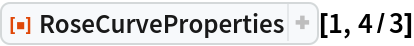Out=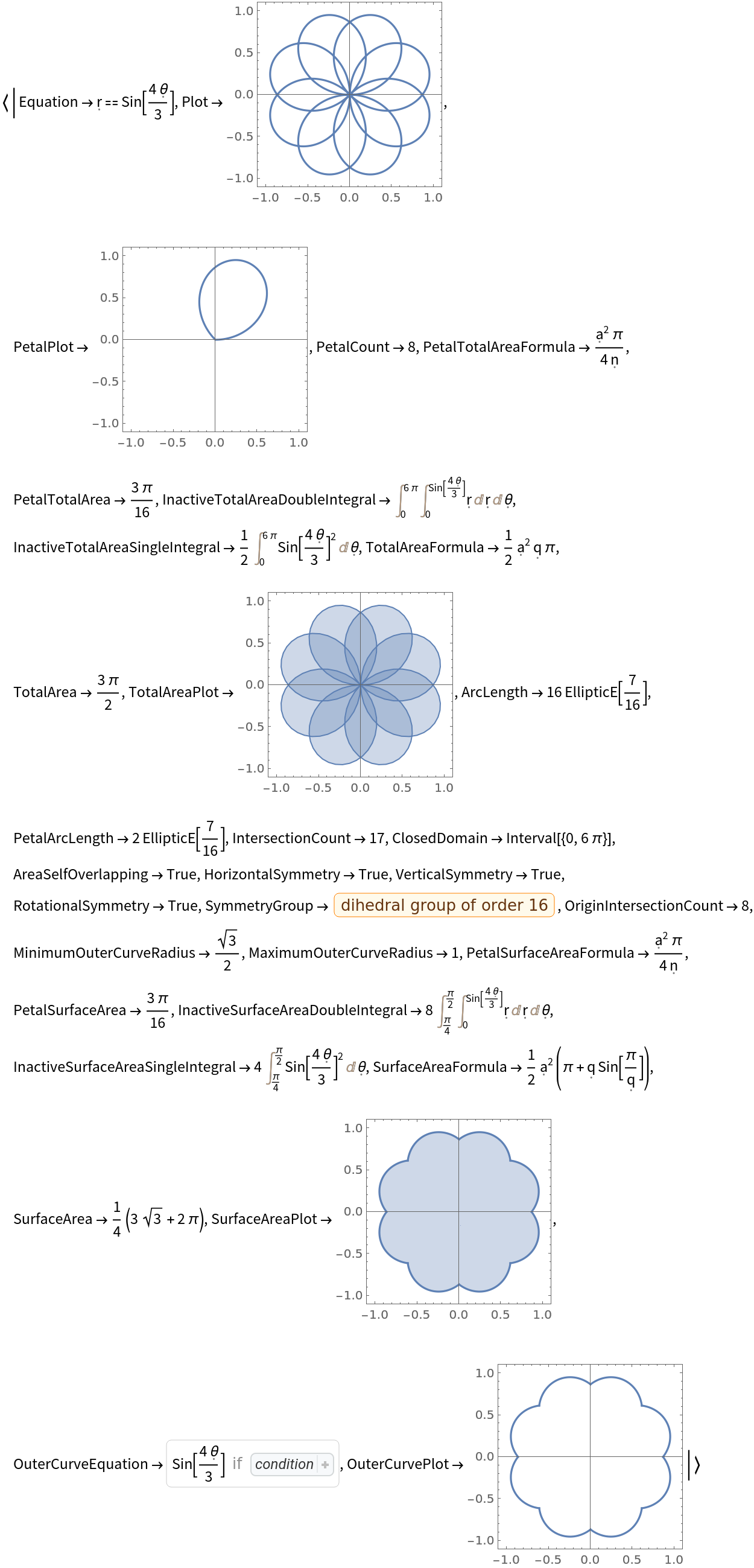Get the number of petals and total area as an Association:

 In:=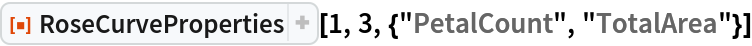Out=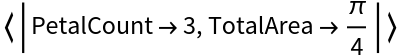Get the same information as a Dataset:

 In:=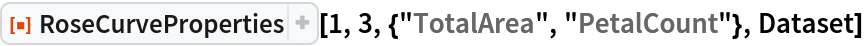Out=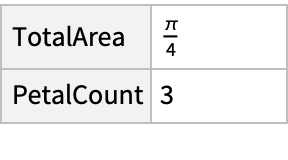### Scope (2)

Find the plot of a rose curve with positive and negative values of a:

 In:=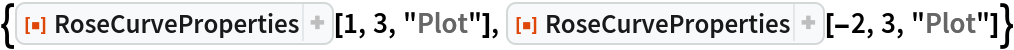Out=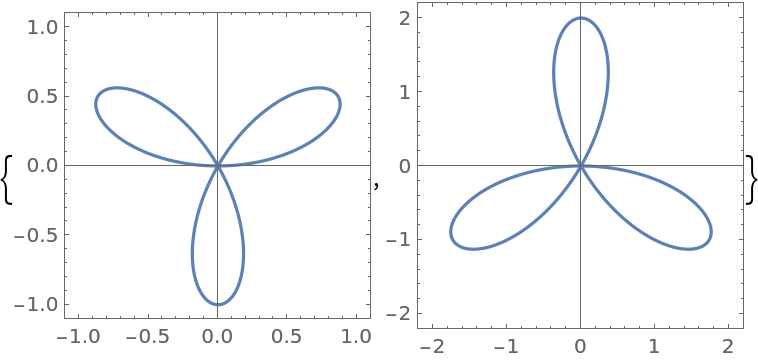Decimal forms of n are acceptable and are rationalized internally to produce exact results:

 In:=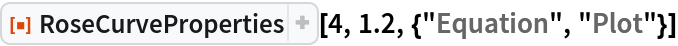Out=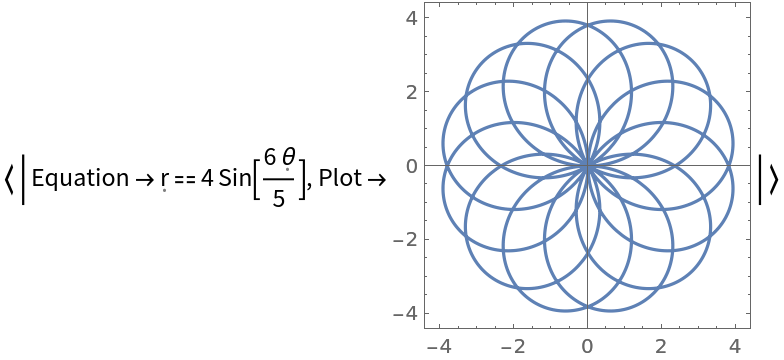### Options (2)

Default PolarPlot options can be used for "Plot", "PetalPlot", and "OuterCurvePlot":

 In:=Out=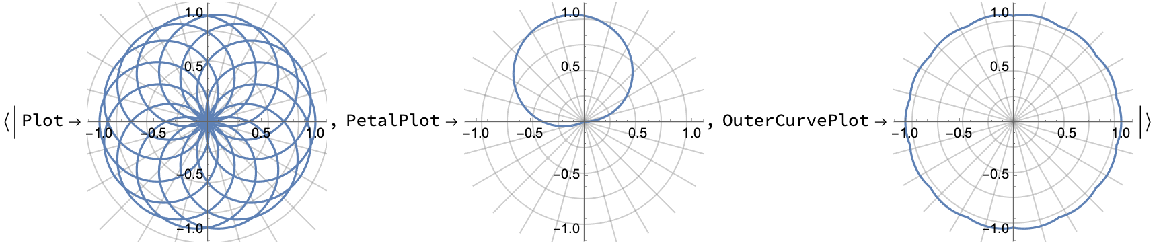Default ParametricPlot options can be used for "TotalAreaPlot":

 In:=Out=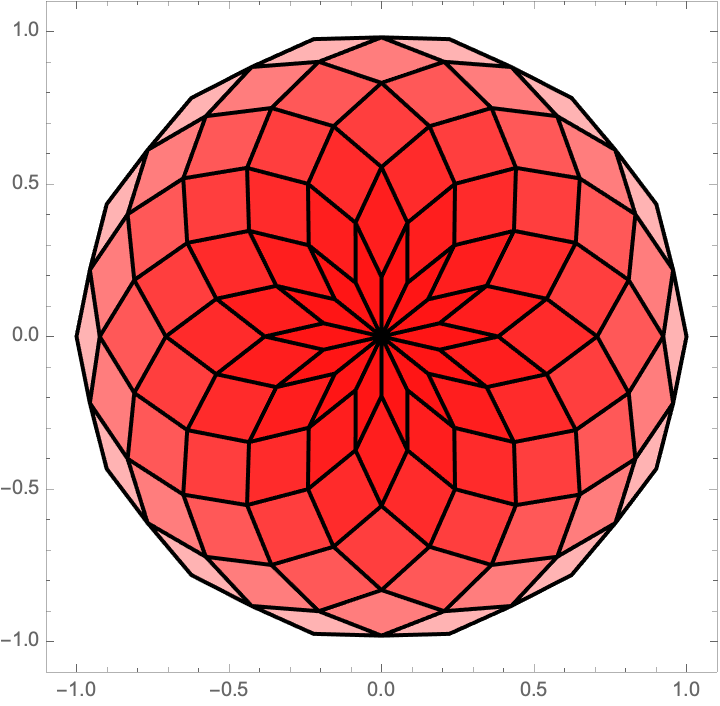### Applications (2)

Depict the different rose curves for n=1/t as t increases:

 In:=Out=Plot the increasing and oscillating number of self-intersections (depending on the parity of t):

 In:=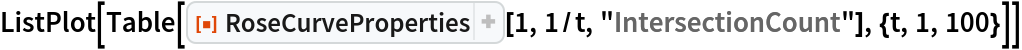Out=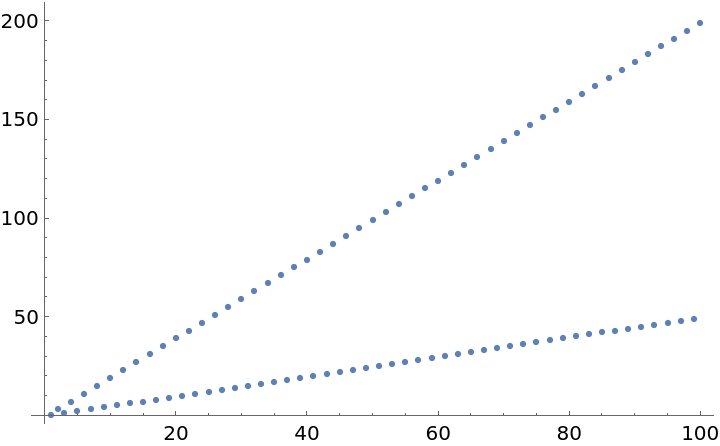Show the change in outer curve plots, which visually approach a circle:

 In:=Out=Plotting the surface area, the rose curve shows the progression towards π:

 In:=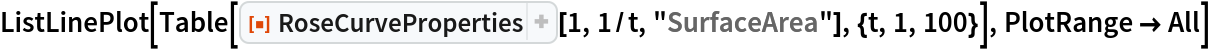Out=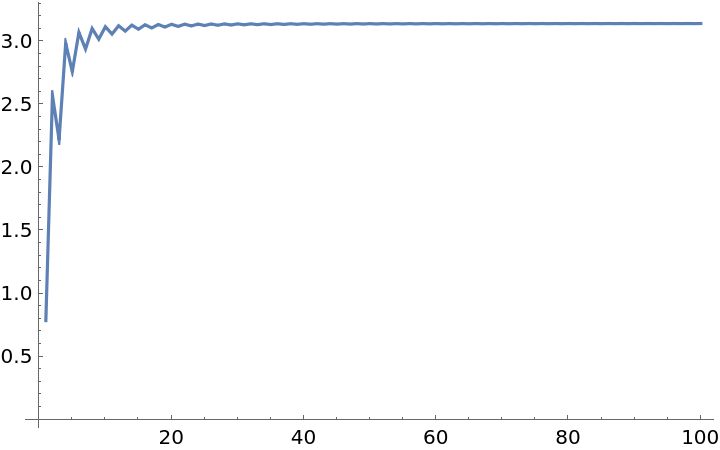Plotting the rose curve and outer curve for n=1/50 explains further why the surface area approaches π:

 In:=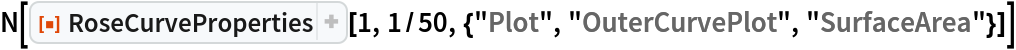Out=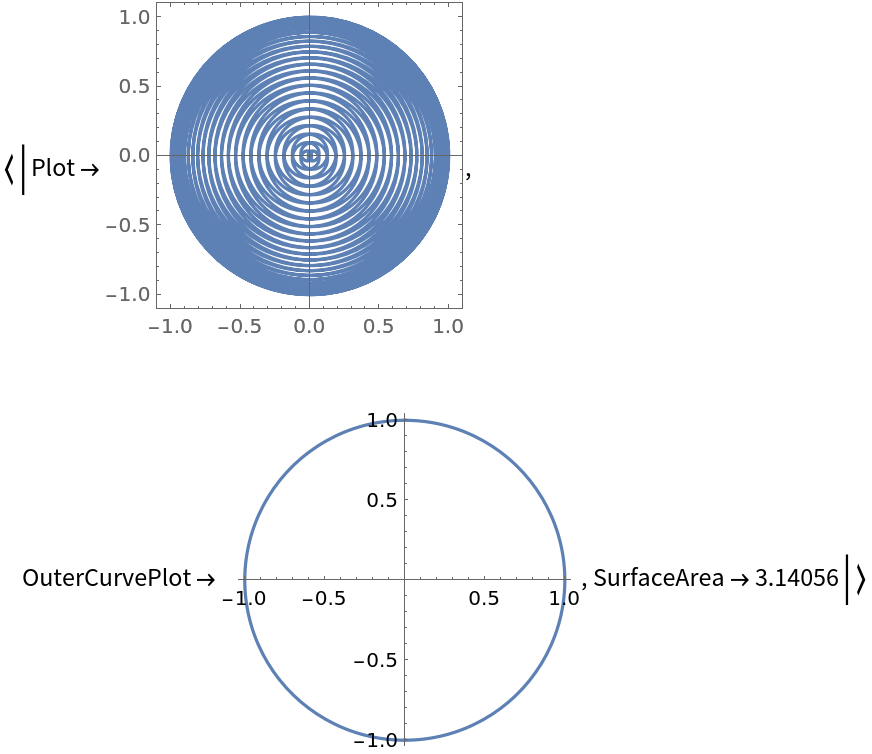Show how a single petal flattens for increasing integer values of n:

 In:=Out=Plot the area of a single petal against the n value:

 In:=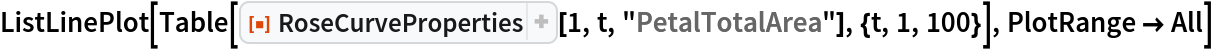Out=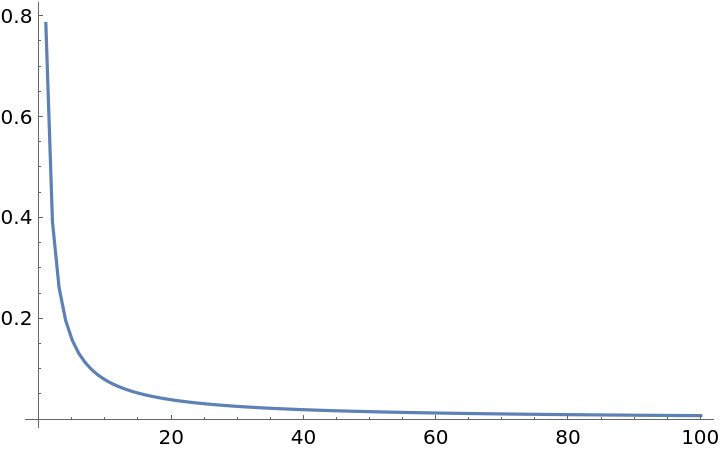The above plot is explained by the "PetalTotalAreaFormula" property:

 In:=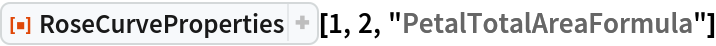Out=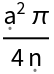### Possible Issues (4)

For the default number of PlotPoints, the area plot may appear choppy:

 In:=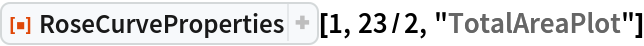Out=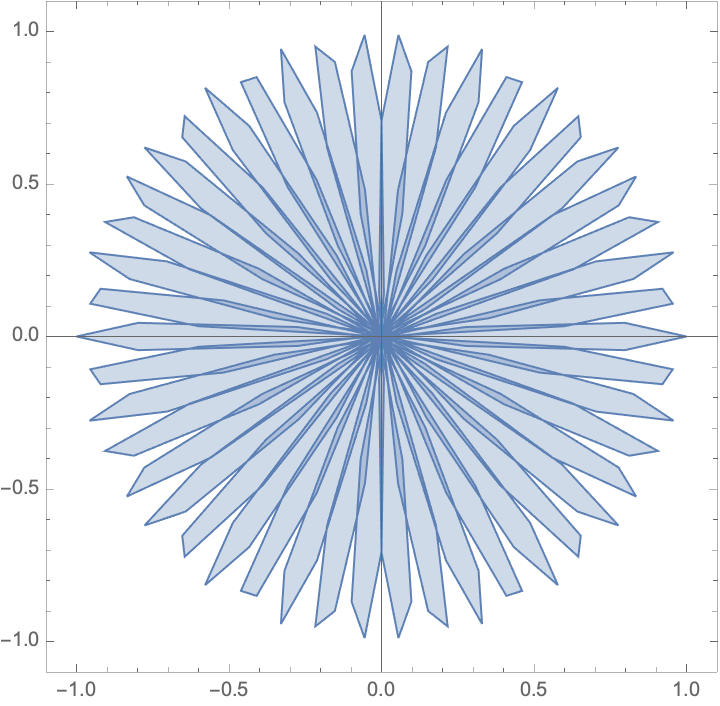Adding a higher value for PlotPoints may take longer, but will produce a smoother and more accurate plot:

 In:=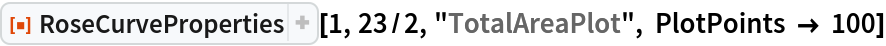Out=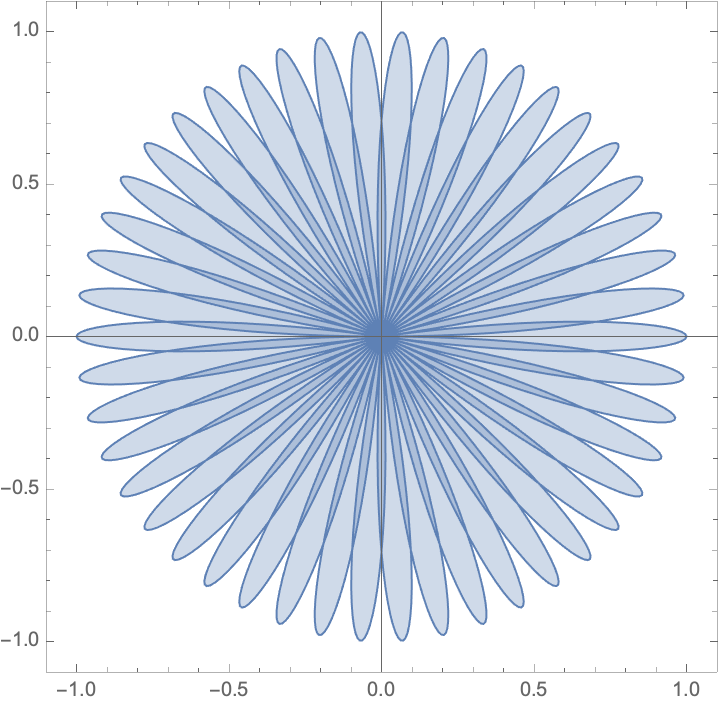For non-numerical values of a, the function is left unevaluated:

 In:=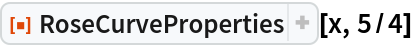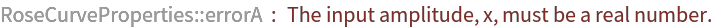Out=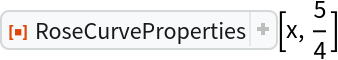For negative, irrational or nonnumerical values of n, the function is left unevaluated:

 In:=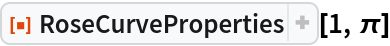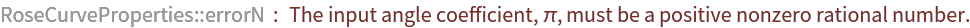Out=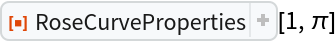When no available property is called, the function is left unevaluated:

 In:=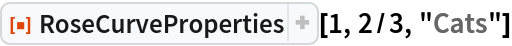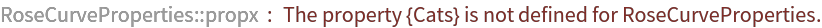Out=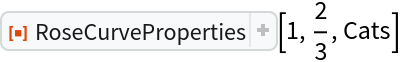### Neat Examples (2)

Visualize the progression of the rose curve plot for integer-valued n:

 In:=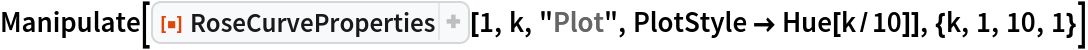Out=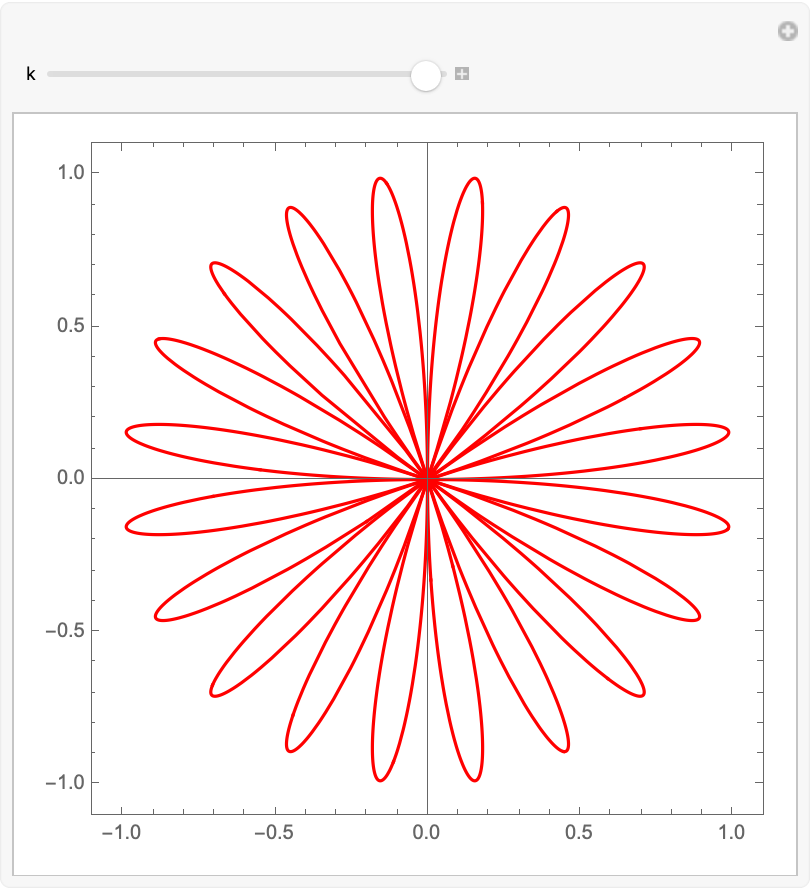Plotting the first 10 rose curves for integer n on one graph:

 In:=Out=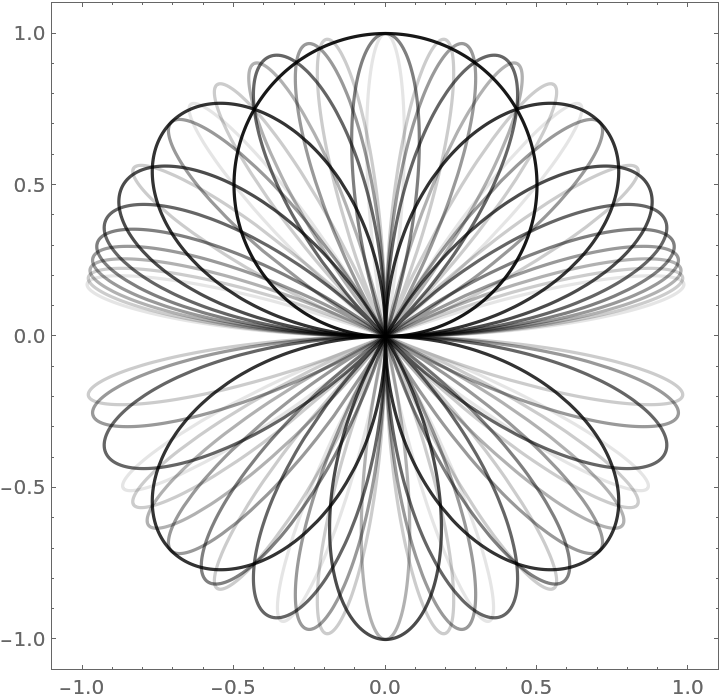Plotting the first 10 rational n (with numerator 1) rose curves on one graph:

 In:=Out=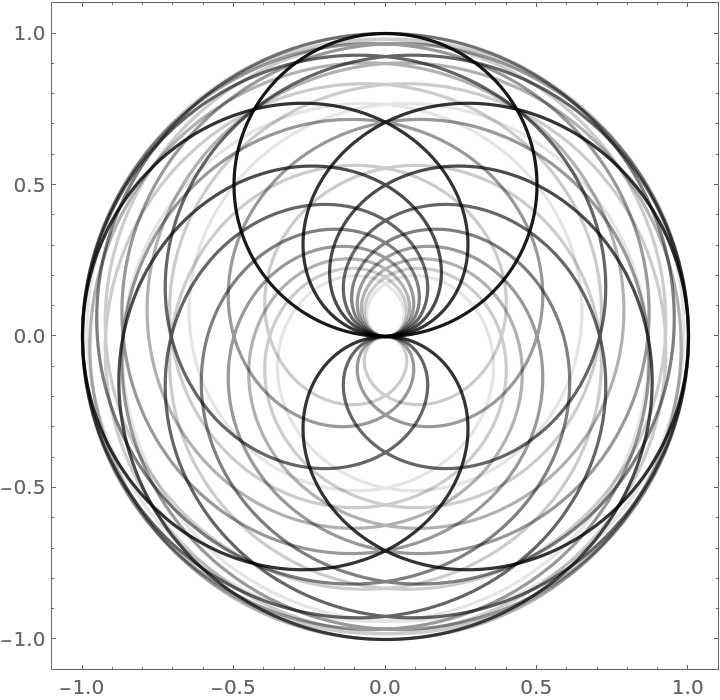Garrett Dubofsky

## Version History

• 1.1.0 – 10 May 2022
• 1.0.0 – 19 October 2021